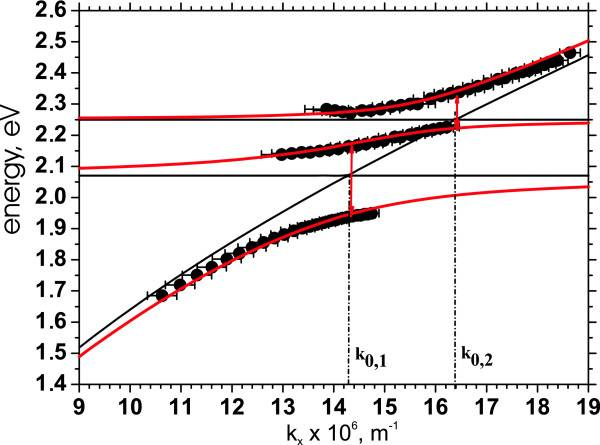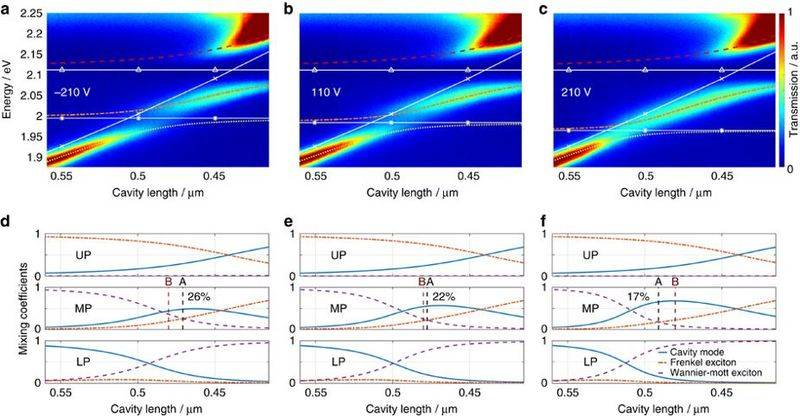# Incorrect Hybrid Polariton Dispersion Results

• A
I am trying to verify the results of the hybrid polariton case with the following hamiltonian, but cannot seem to verify the results in various published papers. Can someone please explain what is wrong and how to get the a similar dispersion graph? I'm solving for the eigenenergies for the matrix below, then solve the cubic using the trigonometric method.

Code:
\begin{pmatrix}
E_c - \lambda & F & W \\
F & E_f - \lambda & 0 \\
W & 0 & E_w - \lambda
\end{pmatrix}

Where Ec is the photon/cavity energy, Ef is the Frenkel exciton energy, and Ew is the Wannier-Mott exciton energy.

The characteristic equation is:
Code:
\begin{equation}
0 = \lambda_n^3 - (E_c + E_f +E_w)\lambda_n^2 + (E_f E_w + E_c E_w + E_c E_f - F^2 - W^2)\lambda_n - E_c E_f E_w +F^2 E_w + W^2 E_f
\end{equation}

Where n = {0,1,2} corresponding to the three hybrid polariton branches. I used the trigonometric formula to solve the cubic for the energy.

Code:
\begin{equation}
\lambda_n = 2 \sqrt{- \frac{p}{3}} \cos \left( \frac{1}{3} \arccos \left( \frac{3q}{2p} \sqrt{- \frac{3}{p}} \right) - \frac{2 \pi n}{3} \right)
\end{equation}

Where p and q are the coefficients of the transformed cubic
Code:
\begin{equation}
\lambda_n^3 + p\lambda_n + q = 0
\end{equation}
.

DrDu
Could it be, that the sign of the terms containing F and W is wrong?

Unfortunately, that isn't the problem (it only worsened the curves).

DrDu
You should give us your references.

The matrix is pretty standard in hybrid polaritonics as well as the 3-oscillator dispersion.Taken from:
Strong coupling between surface plasmon polaritons and Sulforhodamine 101 dye
• March 2012
• Nanoscale Research Letters 7(1):191
• DOI:
• 10.1186/1556-276X-7-191

•Svitlana V Baieva
• Tommi K Hakala
•J. Jussi Toppari

#### Attachments

Cthugha
That is puzzling. You want to calculate the polariton dispersion for two different excitons coupling to a cavity mode, but post a reference for surface plasmons, which have a different dispersion. Most likely you are assuming wrong coupling constants or an incorrect dispersion for the bare particles.

It will be hard to help you, unless you post the exact bare particle dispersions and off-diagonal matrix elements you assume. Is your cavity dispersion parabolic with an effective mass? What is the effective mass you assume? What are the off-diagonal elements? What does your dispersion look like now?

The dispersion for three oscillators like in the SPP case and hybrid polariton case have the same shape. The matrices to be diagonalized are also very similar.

https://www.nature.com/articles/ncomms14097/figures/2#### Attachments

The original post of mine is the correct matrix for the characteristic polynomial.

Cthugha
Well, your equation (2) does not reproduce the bare particle energies in the limit of no coupling (F=0 and W=0), so there is obviously something wrong with it.

This is why I've posted the question. There is something wrong, and I need to help finding it. The hamiltonian is correct and comes from the latter referenced article.

Cthugha
I should have been more clear. Sorry. Could you give us the explicit expressions you get for p and q?Cthugha
Assuming you are not interested in linewidths (imaginary part) at the moment, you are doing it right. This will give you the correct results. A quick check gave me the correct dispersions.
Did you check that:
- hat you remember to convert back to the energies? The roots you calculate this way are for the $\lambda_n$ in the transformed cubic, which is usually called t and not equivalent to your initial $\lambda_n$. You get back to the energy by calculating $E_i=t_i-\frac{b}{3a}$

Thanks! I'll check. Could you tell me what you used for your micro cavity energy?

Cthugha
I just used simple numbers, where I know that the numbers will not create a mess.

The x-value (cavity length, k-value, whatever) goes from 1 to 100. In this range, Ec goes from -50 to 50. Ef=-10. Ew=10. F^2=W^2=10.
Of course these numbers are unphysical, but it is easy to go to meaningful numbers once the equations work well.

If I may, what are you using to graph the dispersion?

Let me ask clearly, you get the cubic (eq 1) for the characteristic polynomial?

Thank you. I have the dispersion with respect to angle of incidence. I'm not sure why my cavity-dispersion wasn't correct when I used cavity length, though. Thanks again!